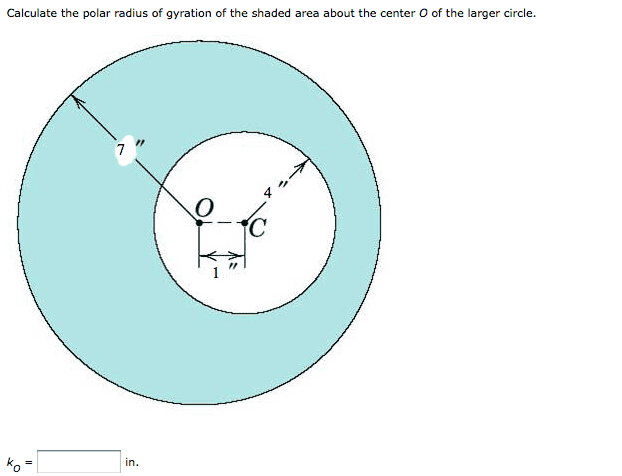# Radius of Gyration and moment of inertia

## Homework Statement## Homework Equations

i guess its r=sqrt(I/A)
where A is the area of the circle thing and I is the moment of intertia.

## The Attempt at a Solution

I guess I'm just having trouble getting I. A is 33pi

what have you tried?

finding the centroid of the shape.

were you able to find that? I think there is a better approach: I = I_outer - I_inner . (be careful that everything is calculated about O).

how do I find I_inner ?

ideasrule
Homework Helper
The moment of inertia of a uniform disk around its center is 1/2MR^2. However, the inner disc (the empty region) is not centered on O, but on C. You have to use the parallel axis theorem to account for this.

so according to that...

1/2*49pi*7^2 [I_outer] - (1/2*16pi*4^2+16*1)[I_inner]=3319

3319/(33pi)=32

sqrt(32)=5.66

And that was right...
thanks guys. I understand it a little better now..